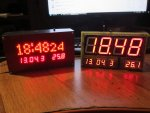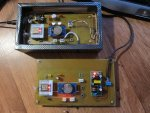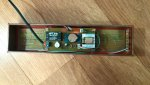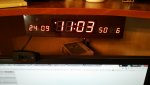# Простые WiFi часы

#### voldemar2005

##### Member
Добрый день!
Представляю Вам свой вариант WiFi часов.Одни часы с использование матричных индикаторов, вторые с большими 1.8" семисегментными индикаторами. Блок индикации собран на MAX7219 или на HT16K33 (Красный 1.2 "4 Цифра 7 Семь Сегментный СВЕТОДИОДНЫЙ Дисплей Часы IIC время for_Arduino оон r3 купить на AliExpress). Управление производится при помощи одной кнопки которая активирует WiFi и дальнейшая настройка производится при помощи Web интерфейса.

Видео работы Web интерфейса:

В архиве находится схема управляющего блока, печатные платы, программа.

#### Вложения

• 580.3 KB Просмотры: 572
Последнее редактирование:

#### planar74

##### New member
Если не секрет, где панель брали? На Али у всех доставка в два раза дороже самой панели.

#### voldemar2005

##### Member
Последнее редактирование:
•planar74 и NutsXXXL

#### Вложения

• 126.4 KB Просмотры: 961
•#### voldemar2005

##### Member
Обновил прошивку, добавил поддержку перепрошивки во воздуху (OTA). Для того чтобы это работало необходимо:

Последнее редактирование:
•romvya

#### romvya

##### New member
Уважаемый @voldemar2005! Собрал ваши часы, Спасибо! то что надо, только один непонятный момент - при отображении времени матрицы перепутаны местами, т.е. (слева на право) четвертая матрица, там где секунды, отображается первой, третья - второй, вторая - третьей, первая, там где часы - последней. Неправильный именно только порядок матриц (при любой смене шрифта), нужно переставить матрицы местами так как описано выше и все будет нормально. Подскажите пожалуйста где подправить код. Матрицы брал такие -Бесплатная Доставка MAX7219 Матричный Модуль Для Arduino Микроконтроллер 4 В Один Дисплей с 5 P Линии купить на AliExpress

#### voldemar2005

##### Member
@romvya Попробуйте а модуле max7219.ino изменить

Код:
``````#ifdef MATRIX
void max_set_row_full( int row, byte *value24, byte value8)
{
if ( row < 0 || row > 7) return;
buf_command = row + 1;
buf_command = value8;
{
buf_command[addr * 2] = row + 1;
}
max_spi_transfer();
}
#else``````

•romvya

#### voldemar2005

##### Member
Обновил первый пост с прошивкой. Из изменений: авто перевод зимнее/летнее время, раз в сутки синхронизация с интернетом, изменение алгоритма автояркости. При обновлении прошивки настройки часов сбросятся на дефолтные.

#### romvya

##### New member
@romvya Попробуйте а модуле max7219.ino изменить

Код:
``````#ifdef MATRIX
void max_set_row_full( int row, byte *value24, byte value8)
{
if ( row < 0 || row > 7) return;
buf_command = row + 1;
buf_command = value8;
{
buf_command[addr * 2] = row + 1;
}
max_spi_transfer();
}
#else``````
Спасибо за быстрый ответ, только сейчас добрался до устройства, сделал как вы сказали - результат тот же вот видео

#### romvya

##### New member
Обновил первый пост с прошивкой. Из изменений: авто перевод зимнее/летнее время, раз в сутки синхронизация с интернетом, изменение алгоритма автояркости. При обновлении прошивки настройки часов сбросятся на дефолтные.
Прошивка что-то не компилируется - ругается на библиотеку time
Arduino: 1.6.8 (Windows 10), Плата:"Generic ESP8266 Module, Serial, 80 MHz, 40MHz, DIO, 115200, 512K (64K SPIFFS), ck, Disabled, None"

libraries\Time\DateStrings.cpp.o: In function `dayStr(unsigned char)':

C:\Users\Roman\Documents\Arduino\libraries\Time/DateStrings.cpp:72: multiple definition of `monthStr(unsigned char)'

sketch\libraries\Time\DateStrings.cpp.o:sketch\libraries\Time/DateStrings.cpp:72: first defined here

libraries\Time\DateStrings.cpp.o: In function `monthShortStr(unsigned char)':

C:\Users\Roman\Documents\Arduino\libraries\Time/DateStrings.cpp:79: multiple definition of `monthShortStr(unsigned char)'

sketch\libraries\Time\DateStrings.cpp.o:sketch\libraries\Time/DateStrings.cpp:79: first defined here

libraries\Time\DateStrings.cpp.o: In function `dayStr(unsigned char)':

C:\Users\Roman\Documents\Arduino\libraries\Time/DateStrings.cpp:86: multiple definition of `dayStr(unsigned char)'

sketch\libraries\Time\DateStrings.cpp.o:sketch\libraries\Time/DateStrings.cpp:86: first defined here

libraries\Time\DateStrings.cpp.o: In function `dayShortStr(unsigned char)':

C:\Users\Roman\Documents\Arduino\libraries\Time/DateStrings.cpp:91: multiple definition of `dayShortStr(unsigned char)'

sketch\libraries\Time\DateStrings.cpp.o:sketch\libraries\Time/DateStrings.cpp:91: first defined here

libraries\Time\Time.cpp.o: In function `setTime(long)':

C:\Users\Roman\Documents\Arduino\libraries\Time/Time.cpp:151: multiple definition of `breakTime(long, tmElements_t&)'

sketch\libraries\Time\Time.cpp.o:sketch\libraries\Time/Time.cpp:151: first defined here

libraries\Time\Time.cpp.o: In function `refreshCache(long)':

C:\Users\Roman\Documents\Arduino\libraries\Time/Time.cpp:42: multiple definition of `refreshCache(long)'

sketch\libraries\Time\Time.cpp.o:sketch\libraries\Time/Time.cpp:42: first defined here

libraries\Time\Time.cpp.o: In function `hour(long)':

C:\Users\Roman\Documents\Arduino\libraries\Time/Time.cpp:53: multiple definition of `hour(long)'

sketch\libraries\Time\Time.cpp.o:sketch\libraries\Time/Time.cpp:53: first defined here

libraries\Time\Time.cpp.o: In function `isPM(long)':

C:\Users\Roman\Documents\Arduino\libraries\Time/Time.cpp:84: multiple definition of `isPM(long)'

sketch\libraries\Time\Time.cpp.o:sketch\libraries\Time/Time.cpp:84: first defined here

libraries\Time\Time.cpp.o: In function `isAM(long)':

C:\Users\Roman\Documents\Arduino\libraries\Time/Time.cpp:76: multiple definition of `isAM(long)'

sketch\libraries\Time\Time.cpp.o:sketch\libraries\Time/Time.cpp:76: first defined here

libraries\Time\Time.cpp.o: In function `hourFormat12(long)':

C:\Users\Roman\Documents\Arduino\libraries\Time/Time.cpp:62: multiple definition of `hourFormat12(long)'

sketch\libraries\Time\Time.cpp.o:sketch\libraries\Time/Time.cpp:62: first defined here

libraries\Time\Time.cpp.o: In function `minute(long)':

C:\Users\Roman\Documents\Arduino\libraries\Time/Time.cpp:92: multiple definition of `minute(long)'

sketch\libraries\Time\Time.cpp.o:sketch\libraries\Time/Time.cpp:92: first defined here

libraries\Time\Time.cpp.o: In function `second(long)':

C:\Users\Roman\Documents\Arduino\libraries\Time/Time.cpp:101: multiple definition of `second(long)'

sketch\libraries\Time\Time.cpp.o:sketch\libraries\Time/Time.cpp:101: first defined here

libraries\Time\Time.cpp.o: In function `day(long)':

C:\Users\Roman\Documents\Arduino\libraries\Time/Time.cpp:110: multiple definition of `day(long)'

sketch\libraries\Time\Time.cpp.o:sketch\libraries\Time/Time.cpp:110: first defined here

libraries\Time\Time.cpp.o: In function `weekday(long)':

C:\Users\Roman\Documents\Arduino\libraries\Time/Time.cpp:119: multiple definition of `weekday(long)'

sketch\libraries\Time\Time.cpp.o:sketch\libraries\Time/Time.cpp:119: first defined here

libraries\Time\Time.cpp.o: In function `month(long)':

C:\Users\Roman\Documents\Arduino\libraries\Time/Time.cpp:128: multiple definition of `month(long)'

sketch\libraries\Time\Time.cpp.o:sketch\libraries\Time/Time.cpp:128: first defined here

libraries\Time\Time.cpp.o: In function `year(long)':

C:\Users\Roman\Documents\Arduino\libraries\Time/Time.cpp:137: multiple definition of `year(long)'

sketch\libraries\Time\Time.cpp.o:sketch\libraries\Time/Time.cpp:137: first defined here

libraries\Time\Time.cpp.o: In function `makeTime(tmElements_t&)':

C:\Users\Roman\Documents\Arduino\libraries\Time/Time.cpp:204: multiple definition of `makeTime(tmElements_t&)'

sketch\libraries\Time\Time.cpp.o:sketch\libraries\Time/Time.cpp:204: first defined here

libraries\Time\Time.cpp.o: In function `setTime(long)':

C:\Users\Roman\Documents\Arduino\libraries\Time/Time.cpp:274: multiple definition of `setTime(long)'

sketch\libraries\Time\Time.cpp.o:sketch\libraries\Time/Time.cpp:274: first defined here

libraries\Time\Time.cpp.o.bss.getTimePtr+0x0): multiple definition of `getTimePtr'

sketch\libraries\Time\Time.cpp.o.bss.getTimePtr+0x0): first defined here

libraries\Time\Time.cpp.o: In function `now()':

C:\Users\Roman\Documents\Arduino\libraries\Time/Time.cpp:250: multiple definition of `now()'

sketch\libraries\Time\Time.cpp.o:sketch\libraries\Time/Time.cpp:250: first defined here

libraries\Time\Time.cpp.o: In function `hour()':

C:\Users\Roman\Documents\Arduino\libraries\Time/Time.cpp:49: multiple definition of `hour()'

sketch\libraries\Time\Time.cpp.o:sketch\libraries\Time/Time.cpp:49: first defined here

libraries\Time\Time.cpp.o: In function `hourFormat12()':

C:\Users\Roman\Documents\Arduino\libraries\Time/Time.cpp:58: multiple definition of `hourFormat12()'

sketch\libraries\Time\Time.cpp.o:sketch\libraries\Time/Time.cpp:58: first defined here

libraries\Time\Time.cpp.o: In function `isAM()':

C:\Users\Roman\Documents\Arduino\libraries\Time/Time.cpp:72: multiple definition of `isAM()'

sketch\libraries\Time\Time.cpp.o:sketch\libraries\Time/Time.cpp:72: first defined here

libraries\Time\Time.cpp.o: In function `isPM()':

C:\Users\Roman\Documents\Arduino\libraries\Time/Time.cpp:80: multiple definition of `isPM()'

sketch\libraries\Time\Time.cpp.o:sketch\libraries\Time/Time.cpp:80: first defined here

libraries\Time\Time.cpp.o: In function `minute()':

C:\Users\Roman\Documents\Arduino\libraries\Time/Time.cpp:88: multiple definition of `minute()'

sketch\libraries\Time\Time.cpp.o:sketch\libraries\Time/Time.cpp:88: first defined here

libraries\Time\Time.cpp.o: In function `second()':

C:\Users\Roman\Documents\Arduino\libraries\Time/Time.cpp:97: multiple definition of `second()'

sketch\libraries\Time\Time.cpp.o:sketch\libraries\Time/Time.cpp:97: first defined here

libraries\Time\Time.cpp.o: In function `day()':

C:\Users\Roman\Documents\Arduino\libraries\Time/Time.cpp:106: multiple definition of `day()'

sketch\libraries\Time\Time.cpp.o:sketch\libraries\Time/Time.cpp:106: first defined here

libraries\Time\Time.cpp.o: In function `weekday()':

C:\Users\Roman\Documents\Arduino\libraries\Time/Time.cpp:115: multiple definition of `weekday()'

sketch\libraries\Time\Time.cpp.o:sketch\libraries\Time/Time.cpp:115: first defined here

libraries\Time\Time.cpp.o: In function `month()':

C:\Users\Roman\Documents\Arduino\libraries\Time/Time.cpp:124: multiple definition of `month()'

sketch\libraries\Time\Time.cpp.o:sketch\libraries\Time/Time.cpp:124: first defined here

libraries\Time\Time.cpp.o: In function `year()':

C:\Users\Roman\Documents\Arduino\libraries\Time/Time.cpp:133: multiple definition of `year()'

sketch\libraries\Time\Time.cpp.o:sketch\libraries\Time/Time.cpp:133: first defined here

libraries\Time\Time.cpp.o: In function `setTime(int, int, int, int, int, int)':

C:\Users\Roman\Documents\Arduino\libraries\Time/Time.cpp:286: multiple definition of `setTime(int, int, int, int, int, int)'

sketch\libraries\Time\Time.cpp.o:sketch\libraries\Time/Time.cpp:286: first defined here

sketch\libraries\Time\Time.cpp.o:sketch\libraries\Time/Time.cpp:303: first defined here

libraries\Time\Time.cpp.o: In function `timeStatus()':

C:\Users\Roman\Documents\Arduino\libraries\Time/Time.cpp:307: multiple definition of `timeStatus()'

sketch\libraries\Time\Time.cpp.o:sketch\libraries\Time/Time.cpp:307: first defined here

libraries\Time\Time.cpp.o: In function `setSyncProvider(long (*)())':

C:\Users\Roman\Documents\Arduino\libraries\Time/Time.cpp:312: multiple definition of `setSyncProvider(long (*)())'

sketch\libraries\Time\Time.cpp.o:sketch\libraries\Time/Time.cpp:312: first defined here

libraries\Time\Time.cpp.o: In function `setSyncInterval(long)':

C:\Users\Roman\Documents\Arduino\libraries\Time/Time.cpp:319: multiple definition of `setSyncInterval(long)'

sketch\libraries\Time\Time.cpp.o:sketch\libraries\Time/Time.cpp:319: first defined here

collect2.exe: error: ld returned 1 exit status

exit status 1
Ошибка компиляции для платы Generic ESP8266 Module.

Этот отчёт будет иметь больше информации с
включенной опцией Файл -> Настройки ->
"Показать подробный вывод во время компиляции"

#### voldemar2005

##### Member
ругается на библиотеку time
скорее всего из папки со скетчем надо убрать все лишнее папки ...

Автор ограничил доступ к видео.

ну а проверить я смогу только завтра, часы у меня на работе ...

#### romvya

##### New member
авто перевод зимнее/летнее время
а если устройство будет отключено во время перевода на летнее время? и включится, например в мае?
Я перевод делал так

Код:
``````// летнее время--------------------------------------------------------------------------------------------------------------

int M, OK ;
// Находим последнее воскресенье марта и последнее воскресенье октября
M = 31 - DayOfWeek(31, 3, tmYearToCalendar(tm.Year));
OK = 31 - DayOfWeek(31, 10, tmYearToCalendar(tm.Year));

// Учёт летнего времни (с последнего воскресенья марта до последнего воскресенья октября)
if  ((((tm.Month > 3) && (tm.Month < 10)) ||
((tm.Month == 3) && (tm.Day > M))   ||
((tm.Month == 3) && (tm.Day == M) && (tm.Hour >= 3))  ||
((tm.Month == 10) && (tm.Day < OK)) ||
((tm.Month == 10) && (tm.Day == OK) && (tm.Hour < 4))))

timeZone = 3; // летнее время

else {
timeZone = 2; // зимнее время
}
//Serial.println(timeZone);

if ((tm.Month == 10) && (tm.Day == OK) && ((tm.Hour > 4) || (tm.Hour < 3)) ||
((tm.Month != 10) && (tm.Day != OK))

)
{ if (var22 == 0) {
var22 = 1; EEPROM.write(3, var22);
Serial.println("var22 EEPROM Write");
}
var22 = 1;  // разрешение для смены timeZone при переводе на час назад
}

if ((zonestatus != timeZone) && var22 == 1)
{
EEPROM.write(2, timeZone);
Serial.println("timeZome EEPROM Write");
Udp.begin(localPort);
delay (2000);
setSyncProvider(getNtpTime);
if (timeStatus() != timeNotSet) //Если время получили успешно,
{
RTC.set(now()); // то записываем данные в RTC
}
else {
if (timeZone == 2) RTC.set(RTC.get() - 3600);
if (timeZone == 3) RTC.set(RTC.get() + 3600);
}
zonestatus = timeZone;
if (timeZone == 2) {
var22 = 0; EEPROM.write(3, var22);
Serial.println("var22=0 EEPROM Write");
}
}

}``````
Код:
``````int DayOfWeek (int day, int month, int ye) // формула определения дня недели
{
unsigned a = (14 - month) / 12;
unsigned y = ye - a;
unsigned m = month + 12 * a - 2;
return (7000 + (day + y + y / 4 - y / 100 + y / 400 + (31 * m) / 12)) % 7;
}``````

#### voldemar2005

##### Member
@romvya вот таким циклом у меня получилось поменять мои часы на ваши, значить и вас должно получится сделать наоборот ).
Код:
``````  for ( int addr = 1, addr1 = MAX_USE - 1; addr < MAX_USE; addr++, addr1--)
{
buf_command[addr1 * 2] = row + 1;
}``````

•romvya

#### romvya

##### New member
должно получится сделать наоборот ).
Ура! Получилось! Спасибо большое. Извините за наглость еще бы пару будильников прикрутить с настройкой по дням недели и вебинтерфейсом.

#### voldemar2005

##### Member
еще бы пару будильников прикрутить с настройкой по дням недели и вебинтерфейсом.
готово! добавил будильники.
Макросом COUNT_ALARM_CLOCK задается количество. При срабатывании высокий уровень на GPIO13 длительностью 1 мин или отключается кратковременным нажатием на кнопку управления.

•andron220v и romvya

#### Сергей_Ф

##### Moderator
Команда форума
@voldemar2005 не возражаете, я ваш код немного переделаю под себя?
Из того, что уже сделал: выбор типа матрицы (порядка элементов) при компиляции, отказ от rtc при желании, обновление через HTTP (ну не нравится мне ОТА), инвертирование (по желанию) сигнала с фотодатчика (плата Witty). С будильниками Вы меня опередили.

Последнее редактирование:

#### Сергей_Ф

##### Moderator
Команда форума
@voldemar2005 вопрос: почему для синхронизации времени с интернетом Вы писали свой код, а не воспользовались библиотекой ntp.h?